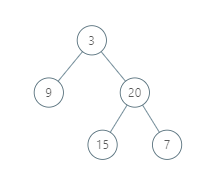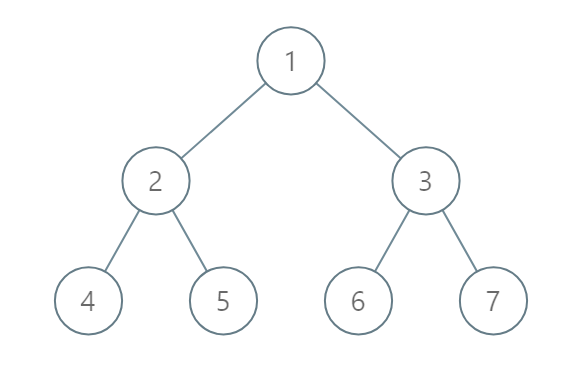# LeetCode: 987. 二叉树的垂序遍历¶

## 1、题目描述¶

X 坐标顺序返回非空报告的列表。每个报告都有一个结点值列表。输入：[3,9,20,null,null,15,7]输入：[1,2,3,4,5,6,7]



• 树的结点数介于 1 和 1000 之间。
• 每个结点值介于 0 和 1000 之间。

## 2、解题思路¶

• 将节点进行坐标编号并放入字典中
• 按照列大小一次从字典中取列表，列表中的顺序按照行排序，同一行按照大小排序
# Definition for a binary tree node.
# class TreeNode:
#     def __init__(self, x):
#         self.val = x
#         self.left = None
#         self.right = None

class Solution:
def verticalTraversal(self, root: TreeNode) -> List[List[int]]:
from collections import defaultdict
mapping = defaultdict(list)

def dfs(node: TreeNode, x, y):
nonlocal mapping
if not node:
return
mapping[y].append((node.val, x))

dfs(node.left, x + 1, y - 1)
dfs(node.right, x + 1, y + 1)

dfs(root, 0, 0)
res = []
for p in sorted(mapping.keys()):
res.append([v for v in sorted(mapping[p], key=lambda x: (x, x))])

return res